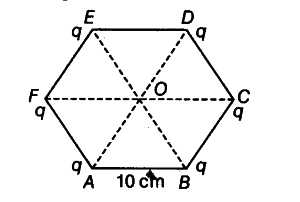# A regular hexagon of side 10 cm has a charge 5µC at each of its vertices. Calculate the potential at the centre of the hexagon

ABCDEF is a regular hexagon of side 10 cm each. At each corner, the charge q — 5µC is placed. O is the centre of the hexagon.Given,AS = BC = CD = DE = EF = FA = 10 cm As, the hexagon has six equilateral mangles, so the distance of centre O from every vertex is 10 cm.
i.e. OA = OB = OC = OD = OE = OF = 10 cm Potential at point O = sum of potential at centre O due to individual point charge